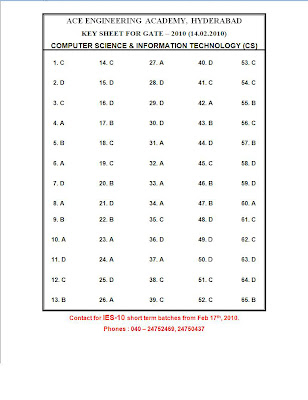## Wednesday, February 17, 2010

### GATE 2010 CS Solutions

Gateforum announced that they will release solutions by 7:30PM on Feb15th, but they didn't. None other coaching institute did. All of them released answer keys only and slept, keeping the students in confusion.
So, I decided, I will post the solutions to selected questions.

If you are just preparing for GATE, you should solve more and more questions. And for that I would recommend GKP Publisher's Question Bank. Although there are some mistakes in the book for solutions to some problems, but I recommend this book solely for the huge collection of problems it has.

Disclaimer: These are my developed solutions. I am a student and I can't guarantee the correctness of the solutions. And yeah, don't think that I solved them all correct. I am not a computer scince wizard either.

Note: Click on the images to enlarge.

First, get the question paper here or here(Thanks Sapan for the second version!)

Now, lets start!

Q-1: I fiddled with it and got the correct answer but didn't mark it cuz of fear of getting it wrong!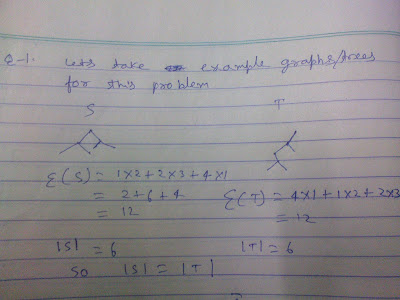Q-2, 3,4,5 I skipped because I had skipped large amount of discrete maths portion and I'm not comfortable with serious topics.

Q-6:
The rightmost thing appearing in this image is P. Read it as PR'. I stripped the R' by mistake while cropping, and overwrote the image.Q-7:
I hit B as explained below.
The refresh operation in a DRAM chip cycles through each row reading the contents of each cell in the row and writing them back.
For the given problem our RAM module will have 32 chips. Pretty easy calculation i.e. (4M*8bits)/(1M*8bits). One refresh cycle refreshes 1 row of each chip being used to construct the module. Thus we require 1K refresh cycles to refresh the wole module by refreshing 1 row of each chip in the module at once.
Hence time required will be 100*(2^10)ns

Q-8:
F 8 7 B is
1111 1000 0111 1011
Multiplying by 8 means multiplying by 2, three times successively. That accounts for 3, 1-bit left shifts i.e. 3-bit left shift.
On performing 3-bit left shift on the number we get
1100 0011 1101 1000
Thats C 3 D 8

Q-9:
Using the equation of multiplexer we have
f=RP'Q'+R'P'Q+R'PQ'+RPQ
=P'(Q'R+R'Q)+P(R'Q'+RQ)
=P'(Q XOR R)+P(Q XNOR R)........(since, A'B+AB' is A XOR B and AB+A'B' is A XNOR B)
=P'(Q XOR R)+P(Q XOR R)'
=P XOR (Q XOR R)
=P XOR Q XOR R..................(since, XOR is associative)

Q-10: I fiddled with it for quite some time and hit C. I believe I was wrong.

Q-11:
I marked B due to some misunderstanding and being in a hurry(dunno why?)!
Proceed to the function call. We have the address of i in p and address of j in q. Now the operation p=q; will transfer j's address in p. The second operation *p=2; will put 2 at j's address. i's address will remain untouched. Hence after returning from the function we get 0 2 as output from the printf function.

Q-12:
I hit D cuz of a calculation mistake.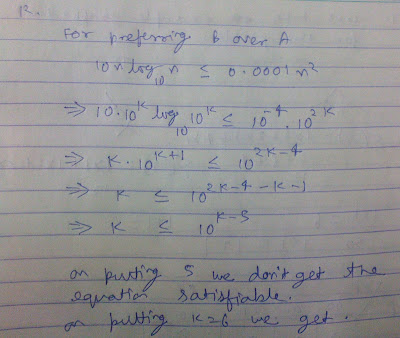Q-13B 14C 15D

Q-16: Seems to be an ambiguous question. A and D are the contenders for being the answer. I hit A.

Q-17:Q-18:
Each node except root in a B+ Tree can hold between T-1 and 2T-1 keys where T is the order of the tree.
In the problem, we have
2T-1=5
=>2T=6
=>T=3
So the min. i.e. T-1 will be
=>T-1=2 hence option B

Q-19 C. Thats pretty obvious

Q-20: I hit A. But thats wrong. 2PL doesn't guarantee freedom from deadlocks. Timestamp ordering does. So B is the right answer.

Q-21: I hit D. I don't have any explanation for it. I had skipped this portion while studying Software Engineering.

Q-22: B is pretty obvious

Q-23: I skipped. I am not comfortable with this thing.

Q-24: 196 is the obvious answer. No need to explain.

Q-25: I hit B but thats wrong. D will be correct. Read Galvin's book.

Q-26: I skipped. I'm not comfortable with probability.

Q-27: Same as above.

Q-28: I drew some example graphs and guessed the answer to be D. And I got it correct!!!!

Q-29: DQ-30: B
For all x, there exists y, there exists t, not F(x,y,t)
For every person x out there, there is a person y, and a time t such that x can't fool y at time t.
Option B i.e. No one can fool everyone all the time expresses the meaning best.

Q-31:
A is correct. This is a simple problem involving De Morgan's law. No need to explain.

Q-32: I skipped. No idea. I ain't an electronics wizard.

Q-33: B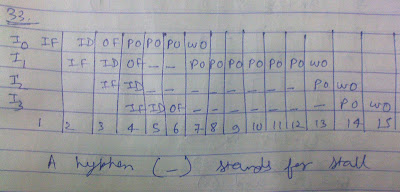Q-34:
I took an example sequence and hit A after applying some unexplainable heuristics. :P

Q-35:
Just follow the recursive call, you'll get 12+(7-(13-(4+(11-(6))))) which is 15.

Q-36:
D is the obvious answer. Moving the last element to the first means setting the pointer of second last element to null and setting head to point to the value of last element(now first) and setting its next pointer point to the to the previous first node.

Q-37:
d=a+b(requires 3 registers. 2 for operands, 1 for result)
e=c+d(a is not used as an operand anywhere ahead so we can overwrite it as e. so, still 3 registers)
f=c+e(we don't need d as an operand, so overwrite it as f. still 3 registers)
b=c+e(we don't need c as operand, so overwrite it as b. still 3 registers)
e=b+f(we don't need b as operand, so overwrite it as e. still 3 of them)
d=5+e(we can overwrite e with the new d. still 3)
return d+f
We're done with 3 registers. But I marked 4 registers. Because in the middle of this whole evaluation I toppled on a variable and needed an extra one.

Q-38: I am uncomfortable with compiler design. I skipped.

Q-39: This one seems to be ambiguous. Consider the string 1010011. It has even number of ones. But none of the given regular expressions can generate it. I didn't mark anything.

Q-40: A CFG can keep the count of 1 thing. D seems to be the obvious answer. I hit C. Even I don't know why!!!!

Q-41: I hit B because I thought, to accept a substring we need equal or lesser number of states. Dunno whether I am write or wrong, but everyone says I am wrong. Rest in peace.

Q-42:
I hit C cuz of a confusion. *damn*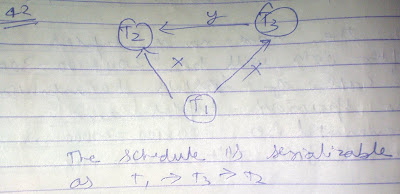Q-43:Q-44:
Go through the test conditions T1 through T4.
You'll deduce that:
T1->S1
T2->S3
T3->S1
T4->S2,S4

so T1,T2,T4->{S1.S2,S3,S4)

Q-45 and 46 I skipped because I am scared of these things.

Q-47: D is the obvious answer. No need to explain. Just bitwise AND 255.255.255.224 to 10.105.1.113 and 10.105.1.91 and you will get different network addresses.

Q-48 and 49: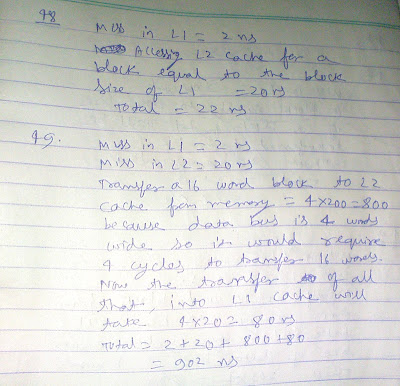Q-50 and 51:
Label the rows and columns of the matrix as 0,1,2,3,4 and draw the graph. Apply Kruskal's algorithm on Q-50 skipping the second 1 weight edge.Edit: For Q-51 the required path is 1-0-4-2 and that is 8 units.. I made the same mistake(rather blunder) in exam hall as well which will set me back by -2.66 marks andaround 150-250 rank positions.

Q-52: Pretty easy. Ans. C

Q-53: I made some heuristics and guessed the ans. to be C 30. And that seems to be right! Al the coaching institutes converge at that answer!

Q-54 and 55: I think I got both of them wrong. I hit B and D respectively.

## Monday, February 15, 2010

### GATE - 2010

If you are just preparing for GATE, you should solve more and more questions. And for that I would recommend GKP Publisher's Question Bank. Although there are some mistakes in the book for solutions to some problems, but I recommend this book solely for the huge collection of problems it has.

Graduate Aptitude Test in Engineering - GATE 2010 was conducted all over India yesterday. I too appeared in the CS paper. The various answer keys provided by different coaching institutes are all differing in their answers. Roughly I'll be getting 45 marks. The results will be declared on March 15th 2010. Here I'm posting all the available answer keys for now.